## Permutation

The rearrangement of elements in a set into a One-to-One correspondence with itself, also called an Arrangement or Order. The number of ways of obtainingordered outcomes from a permutation ofelements is(1)

whereisFactorial andis a Binomial Coefficient. The total number of permutations forelements is given by.

A representation of a permutation as a product of Cycles is unique (up to the ordering of the cycles). An example of a cyclic decomposition is (,), corresponding to the permutations (,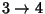,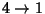) and (), which combine to give.

Any permutation is also a product of Transpositions. Permutations are commonly denoted in Lexicographic or Transposition Order. There is a correspondence between a Permutation and a pair of Young Tableaux known as the Schensted Correspondence.

The number of wrong permutations ofobjects is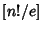whereis the Nint function. A permutation ofordered objects in which no object is in its natural place is called a Derangement (or sometimes, a Complete Permutation) and the number of such permutations is given by the Subfactorial.

Using(2)

withgives(3)

so the number of ways of choosing 0, 1, ..., orat a time is.

The set of all permutations of a set of elements 1, ...,can be obtained using the following recursive procedure(4)(5)

Let the set of Integers 1, 2, ...,be permuted and the resulting sequence be divided into increasing Runs. Asapproaches Infinity, the average length of theth Run is denoted. The first few values are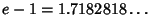(6)(7)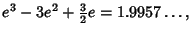(8)

where e is the base of the Natural Logarithm (Knuth 1973, Le Lionnais 1983).

See also Alternating Permutation, Binomial Coefficient, Circular Permutation, Combination, Complete Permutation, Derangement, Discordant Permutation, Eulerian Number, Linear Extension, Permutation Matrix, Subfactorial, Transposition

References

Bogomolny, A. Graphs.'' http://www.cut-the-knot.com/do_you_know/permutation.html.

Conway, J. H. and Guy, R. K. Arrangement Numbers.'' In The Book of Numbers. New York: Springer-Verlag, p. 66, 1996.

Dickau, R. M. Permutation Diagrams.'' http://forum.swarthmore.edu/advanced/robertd/permutations.html.

Knuth, D. E. The Art of Computer Programming, Vol. 1: Fundamental Algorithms, 2nd ed. Reading, MA: Addison-Wesley, 1973.

Kraitchik, M. The Linear Permutations ofDifferent Things.'' §10.1 in Mathematical Recreations. New York: W. W. Norton, pp. 239-240, 1942.

Le Lionnais, F. Les nombres remarquables. Paris: Hermann, pp. 41-42, 1983.

Ruskey, F. Information on Permutations.'' http://sue.csc.uvic.ca/~cos/inf/perm/PermInfo.html.

Sloane, N. J. A. Sequence A000142/M1675 in An On-Line Version of the Encyclopedia of Integer Sequences.'' http://www.research.att.com/~njas/sequences/eisonline.html and Sloane, N. J. A. and Plouffe, S. The Encyclopedia of Integer Sequences. San Diego: Academic Press, 1995.